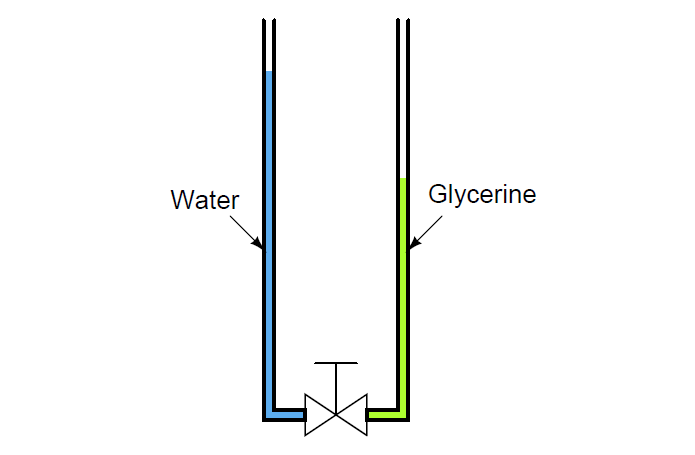# Calculate the Height of Glycerine

Question 1:

Calculate the height of glycerine ( = 78.6 lb/ft3) in a vertical tube if there is 21 PSI of hydrostatic pressure at the bottom of the tube:Glycerine height = __________ ft

Also, calculate the height of castor oil ( = 60.5 lb/ft3) necessary to generate the exact same amount of pressure:

Castor oil height = __________ ft

Glycerine height = 38.46 ft

Castor oil height = 49.96 ft

Question 2:

The following illustration shows a rather strange manometer, one with two different liquids inside, coupled by a hand valve:If the two columns of liquid are just right, they will remain at their respective (different) heights when the valve is opened.

Unlike a normal manometer where the two liquid columns always equalize to the same height when vented, this manometer is “content” to rest at different heights.

Explain why. Also, calculate two possible heights that will balance each other, given the liquids of water and glycerine.

19 inches of water and 15 inches of glycerine will balance one another in this manometer. These are not the only column heights that will self-balance.

In fact, any ratio of 19:15 will work because the ratio of water’s density to glycerine’s density is 15:19.